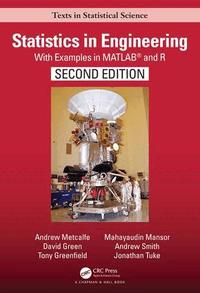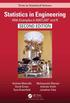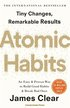Fler böcker inom
Format
Inbunden (Hardback)
Språk
Engelska
Antal sidor
810
Utgivningsdatum
2019-01-29
Upplaga
2 ed
Förlag
Taylor & Francis Inc
Medarbetare
Tuke, Jonathan (förf)
Illustrationer
100 Illustrations, black and white
Dimensioner
254 x 178 x 43 mm
Vikt
1612 g
Antal komponenter
1
Komponenter
68:B&W 7 x 10 in or 254 x 178 mm Case Laminate on White w/Gloss Lam
ISBN
9781439895474

### Du kanske gillar# Statistics in Engineering

With Examples in MATLAB (R) and R, Second Edition

Inbunden Engelska, 2019-01-29
1124
• Skickas inom 7-10 vardagar.
• Gratis frakt inom Sverige över 199 kr för privatpersoner.
Finns även som
632
632
• Skickas inom 10-15 vardagar
613
Engineers are expected to design structures and machines that can operate in challenging and volatile environments, while allowing for variation in materials and noise in measurements and signals. Statistics in Engineering, Second Edition: With Examples in MATLAB and R covers the fundamentals of probability and statistics and explains how to use these basic techniques to estimate and model random variation in the context of engineering analysis and design in all types of environments. The first eight chapters cover probability and probability distributions, graphical displays of data and descriptive statistics, combinations of random variables and propagation of error, statistical inference, bivariate distributions and correlation, linear regression on a single predictor variable, and the measurement error model. This leads to chapters including multiple regression; comparisons of several means and split-plot designs together with analysis of variance; probability models; and sampling strategies. Distinctive features include: All examples based on work in industry, consulting to industry, and research for industry Examples and case studies include all engineering disciplines Emphasis on probabilistic modeling including decision trees, Markov chains and processes, and structure functions Intuitive explanations are followed by succinct mathematical justifications Emphasis on random number generation that is used for stochastic simulations of engineering systems, demonstration of key concepts, and implementation of bootstrap methods for inference Use of MATLAB and the open source software R, both of which have an extensive range of statistical functions for standard analyses and also enable programing of specific applications Use of multiple regression for times series models and analysis of factorial and central composite designs Inclusion of topics such as Weibull analysis of failure times and split-plot designs that are commonly used in industry but are not usually included in introductory textbooks Experiments designed to show fundamental concepts that have been tested with large classes working in small groups Website with additional materials that is regularly updated Andrew Metcalfe, David Green, Andrew Smith, and Jonathan Tuke have taught probability and statistics to students of engineering at the University of Adelaide for many years and have substantial industry experience. Their current research includes applications to water resources engineering, mining, and telecommunications. Mahayaudin Mansor worked in banking and insurance before teaching statistics and business mathematics at the Universiti Tun Abdul Razak Malaysia and is currently a researcher specializing in data analytics and quantitative research in the Health Economics and Social Policy Research Group at the Australian Centre for Precision Health, University of South Australia. Tony Greenfield, formerly Head of Process Computing and Statistics at the British Iron and Steel Research Association, is a statistical consultant. He has been awarded the Chambers Medal for outstanding services to the Royal Statistical Society; the George Box Medal by the European Network for Business and Industrial Statistics for Outstanding Contributions to Industrial Statistics; and the William G. Hunter Award by the American Society for Quality.

## Passar bra ihop

1.2. +
3.De som köpt den här boken har ofta också köpt Atomic Habits av James Clear (häftad).

## Kundrecensioner

Har du läst boken? Sätt ditt betyg »

## Recensioner i media

"Statistics in Engineering: With Examples in MATLAB and R" is an ideal and unreservedly recommended textbook for college and university library collections." ~John Burroughs, Reviewer's Bookwatch "Distinctive features of this new second edition of Statistics in Engineering iinclude: All examples being based on work in industry, consulting to industry, and research for industry; Emphasis on probabilistic modeling including decision trees, Markov chains and processes, and structure functions; Intuitive explanations are followed by succinct mathematical justifications; Emphasis on random number generation that is used for stochastic simulations of engineering systems, demonstration of key concepts, and implementation of bootstrap methods for inference; Use of MATLAB and the open source software R, both of which have an extensive range of statistical functions for standard analyses and also an extensive range of statistical functions for standard analyses and also enable programming of specific applications; Use of multiple regression for times series models and analysis of factorial and central composite for time series models and analysis of factorial and central composite designs; Inclusion of topics such as Weibull analysis of failure times and split-plot designs that are commonly used in industry but are not usually included in introductory textbooks: Experiments designed to show fundamental concepts that have been tested with large classes working in small groups." ~Midwest Book Review

## Övrig information

Andrew Metcalfe, David Green, Andrew Smith, and Jonathan Tuke have taught probability and statistics to students of engineering at the University of Adelaide for many years and have substantial industry experience. Their current research includes applications to water resources engineering, mining, and telecommunications. Mahayaudin Mansor worked in banking and insurance before teaching statistics and business mathematics at the Universiti Tun Abdul Razak Malaysia and is currently a researcher specializing in data analytics and quantitative research in the Health Economics and Social Policy Research Group at the Australian Centre for Precision Health, University of South Australia. Tony Greenfield, formerly Head of Process Computing and Statistics at the British Iron and Steel Research Association, is a statistical consultant. He has been awarded the Chambers Medal for outstanding services to the Royal Statistical Society; the George Box Medal by the European Network for Business and Industrial Statistics for Outstanding Contributions to Industrial Statistics; and the William G. Hunter Award by the American Society for Quality. Visit their website here: http://www.maths.adelaide.edu.au/david.green/BookWebsite/

## Innehållsförteckning

I Foundations Why Understand Statistics? Introduction Using the book Software Probability and Making Decisions Introduction Random digits Concepts and uses Generating random digits Pseudo random digits Defining probabilities Defining probabilities {Equally likely outcomes Defining probabilities {relative frequencies Defining probabilities {subjective probability and expected monetary value Axioms of Probability The addition rule of probability Complement Conditional probability Conditioning on information Conditional probability and the multiplicative rule Independence Tree diagrams Bayes' theorem Law of total probability Bayes' theorem for two events Bayes' theorem for any number of events Decision trees Permutations and combinations Simple random sample Summary Notation Summary of main results MATLAB and R commands Exercises Graphical Displays of Data and Descriptive Statistics Types of variables Samples and populations Displaying data Stem-and-leaf plot Time series plot Pictogram Pie chart Bar chart Rose plot Line chart for discrete variables Histogram and cumulative frequency polygon for continuous variables Pareto chart Numerical summaries of data Population and sample Measures of location Measures of spread Box-plots Outlying values and robust statistics Outlying values Robust statistics Grouped data Calculation of the mean and standard deviation for discrete data Grouped continuous data [mean and sd for grouped continuous data] Mean as center of gravity Case study of wave stress on offshore structure Shape of distributions Skewness Kurtosis Some contrasting histograms Multivariate data Scatter plot Histogram for bivariate data Parallel coordinates plot Descriptive time series Definition of time series Missing values in time series Decomposition of time series Centered moving average Additive monthly model Multiplicative monthly model Seasonal adjustment Forecasting Index numbers Summary Notation Summary of main results MATLAB and R commands Exercises Discrete Probability Distributions Discrete random variables Definition of a discrete probability distribution Expected value Bernoulli trial Binomial distribution Introduction Defining the binomial distribution A model for conductivity Random deviates from binomial distribution Fitting a binomial distribution Hypergeometric distribution Defining the hypergeometric distribution Random deviates from the hypergeometric distribution Fitting the hypergeometric distribution Negative binomial distribution The geometric distribution Defining the negative binomial distribution Applications of negative binomial distribution Fitting a negative binomial distribution Random numbers from a negative binomial distribution Poisson process Defining a Poisson process in time Superimposing Poisson processes Spatial Poisson Process Modifications to Poisson processes Poisson distribution Fitting a Poisson distribution Times between events Summary Notation Summary of main results MATLAB and R commands Exercises Continuous Probability Distributions Continuous probability distributions Definition of a continuous random variable Definition of a continuous probability distribution Moments of a continuous probability distribution Median and mode of a continuous probability distribution Parameters of probability distributions Uniform distribution Definition of a uniform distribution Applications of the uniform distribution Random deviates from a uniform distribution Distribution of F(X) is uniform Fitting a uniform distribution Exponential distribution Definition of an exponential distributi